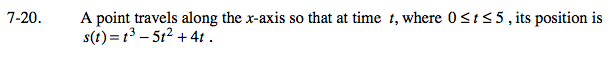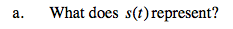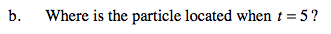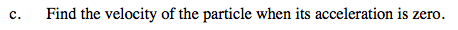### Home > CALC > Chapter 7 > Lesson 7.1.2 > Problem7-20

7-20.
1. A point travels along the x-axis so that at time t, where 0 ≤ t ≤ 5, its position is s(t) = t3 − 5t2 + 4t. Homework Help ✎

1. What does s(t) represent?

2. Where is the particle located when t = 5?

3. Find the velocity of the particle when its acceleration is zero.The point's position along the x-axis.Calculate s(5).Velocity is the derivative of position, and acceleration is the derivative of velocity.